# Optimization options

## Introduction

In the data tree of Flux the node Solver > Optimization > Optimization Options allows the user to set the different options for the optimization algorithm.

The table in the next section summarizes all the optimization options.

## General optimization options

Option Required informations
Optimization tolerance (in percent) Stops the optimization process when the objective value varies (d) less than the tolerance value between two iterations.Default value = 0.5%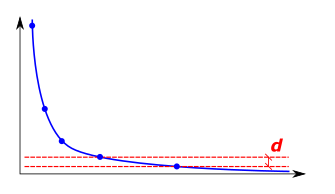Open a Flux with the final design when optimization is done
• Yes
• No
Maximum number of iterations Stops the optimization process when the maximum number of iterations is reached if the convergence was not met.Default value = 30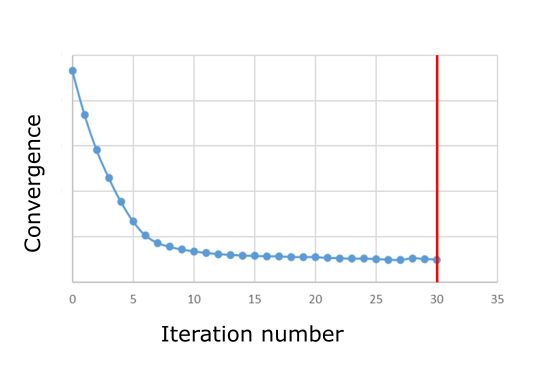Number of iterations before remeshing Defines the number of iteration to complete before remeshing the design parts. Allows to improve the optimization by adding nodes and elements when these become of too poor quality following displacements.
Move limit Define an area where the nodes can move, this area may be defined by:
• A relative factor named r multiplied by the average element mesh size.Default value of the relative factor= 5.0or,
• An absolute move limit (in meter).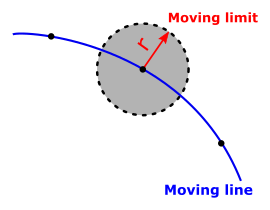Sensbilities filter Define an area where the nodes will move in an average direction composed of each node directions, this area may be defined as:
• A relative factor named r multiplied by the average element mesh size.Default value of the relative factor= 4.0or,
• An absolute radius (in meter).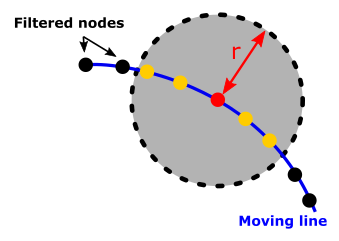Tolerance for the barriers This tolerance is used to control how close the lines of the final design can be. 0.5 meaning half of a mesh size.Default value = 0.1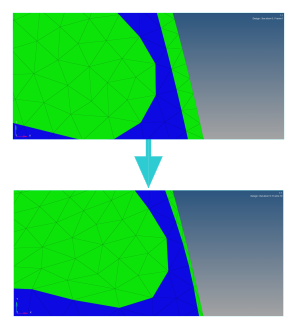## Shape optimization options

Options Required informations
Maximum number of iterations Stops the optimization process when the maximum number of iterations is reached if the convergence was not met.Default value = 30Number of iterations before remeshing Defines the number of iteration to complete before remeshing the design parts. Allows to improve the optimization by adding nodes and elements when these become of too poor quality following displacements.
Move limit Define an area where the nodes can move, this area may be defined by:
• A relative factor named r multiplied by the average element mesh size.Default value of the relative factor= 5.0or,
• An absolute move limit (in meter).Sensbilities filter Define an area where the nodes will move in an average direction composed of each node directions, this area may be defined as:
• A relative factor named r multiplied by the average element mesh size.Default value of the relative factor= 4.0or,
• An absolute radius (in meter).Tolerance for the barriers This tolerance is used to control how close the lines of the final design can be. 0.5 meaning half of a mesh size.Default value = 0.1